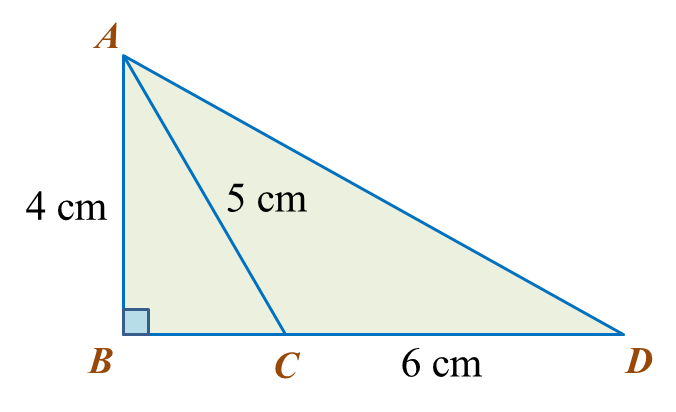# 13.2.1 Pythagoras’ Theorem, PT3 Focus Practice

    
       
        
13.2.1 Pythagoras’ Theorem, PT3 Focus Practice
         

         
Question 1:In the diagram, ABC is a right-angled triangle and BCD is a straight line. Calculate the length of AD, correct to two decimal places.
         
  
        

     Solution:
         
In ∆ ABC,
         
BC= 52 – 42
         
 = 25 – 16
         
 = 9
         
BC = √9
         
= 3 cm
         
         

     In ∆ ABD,
         
BD = BC + CD
         
= 3 + 6
         
= 9
         
         
 = 16 + 81
         
 = 97
         
         
= 9.849
         
= 9.85 cm
    
    
  
     
    
       
        
Question 2:
         
Diagram below shows a triangle ACD and ABC is a straight line.Calculate the length, in cm, of AD.
         
  
        

     Solution:
         
In ∆ DBC,
         
BC= 252 – 242
         
 = 625 – 576
         
 = 49
         
BC = √49
         
= 7 cm
         
AB = 17 – 7 = 10 cm
         
         

     In ∆ DAB,
         
         
 = 100 + 576
         
 = 676